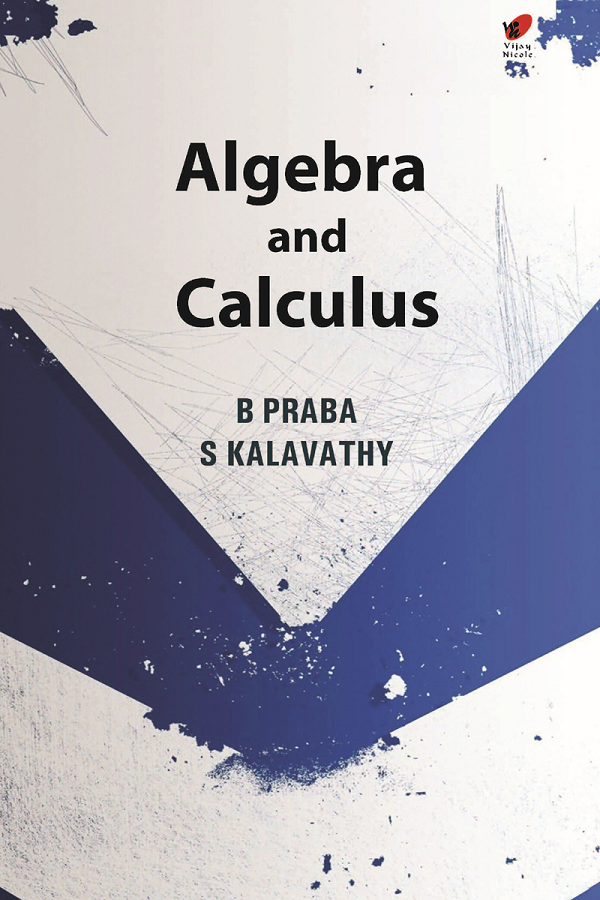Algebra and Calculus
Author(s):
Subject(s):
ISBN 9789394681309
Published Date
Pages 622
Description

Designed to cater to the requirements of first year engineering students, this book completely covers the prescribed syllabus of SSN College of Engineering, (An Autonomous Institution Affilliated to Anna University, Chennai), Kalavakkam, Chennai.

Cover
Title Page
Contents
Preface
UNIT - I TRIGONOMETRIC SERIES
Introduction
De Moivre’s Theorem
Roots of a Complex Number
Expansion of sin nθ, cos nθ and tan nθ in powers of sin θ, cos θ and tan θ
Addition Formulae for Any Number of Angles
Complex Function
Circular Functions of a Complex Variable
Hyperbolic Functions
Inverse Hyperbolic Functions
Logarithmic Function
UNIT - II MATRICES
Introduction
Rank of a Matrix
Simultaneous Linear Equations
Rouche’s Theorem
Homogeneous System of Linear Equations
Linear Independence and Dependence of Vectors
Eigen Values and Eigen Vectors
Introduction
Properties of Eigen Values and Eigen Vectors
Cayley-Hamilton Theorem
Similar Matrices
Diagonalisation of a Matrix
Orthogonal Matrix
Properties
Simultaneous Reduction of Two Quadratic Forms
Canonical Forms of Conics
UNIT - III DIFFERENTIAL CALCULUS
Curvature
Definition of Curvature
Curvature of a Circle
Definition
Cartesian Form of Radius of Curvature
Parametric Form of Radius of Curvature
Polar Form of Radius of Curvature
Centre and Circle of Curvature
Definition
The Centre of Curvature
Equation of Circle of Curvature
Involutes and Evolutes
Evolute
Involute
Procedure for the Equation to the Evolute of the Curve y = f (x)
Properties of Evolutes
Geometrical Method of Proof
Envelopes
Family of Curves
Envelope—Definition
Analytical Method of Finding the Equation of Envelope
Envelope of Two Parameter Family
Property of Envelope
Examples of Property of Envelope
Evolute as the Envelope of Normals
UNIT - IV FUNCTIONS OF SEVERAL VARIABLES
Partial Derivatives
Definition
Total Differentiation
Derivatives of Implicit Functions
Euler’s Theorem for Homogeneous Functions
Total Derivatives
Functions of Functions and Implicit Functions
Taylor’s Theorem
Jacobians
Properties
Maxima and Minima of Functions of Two variables
Definition—Maximum
Definition—Minimum
Working Rule for Finding the Extreme Values of f(x,y)
Constrained Maxima and Minima
Lagrange’s Method of UndeterminedMultipliers
Differentiation under the Integral Sign
UNIT - VINTEGRAL CALCULUS
Introduction
Double Integrals
Evaluation of Double Integrals
Beta and Gamma Function
Relation between Beta and Gamma Functions
Area as Double Integral
Changing the Order of Integration
Application of Beta Gamma Functions to Multiple Integrals
Transformation from Cartesian Coordinates to Polar Coordinates
Triple Integrals
Evaluation of Triple Integrals
Volume as Triple Integral
Solved Question Bank
Index

B Praba is presently Associate Professor, Department of Mathematics, SSN College of Engineering, Chennai. She has 22 years of research and teaching experience and has written books on Discrete Mathematics, Statistics, Random Process and Queuing Theory and Statistics for Management. She has obtained her Ph.D., from Ramanujan Institute for Advanced Study in Mathematics, Chennai.

S Kalavathy is Professor, Department of Mathematics, RMD Engineering College, Chennai. She has over 23 years of experience in teaching Mathematics and has written several books including best sellers on Engineering Mathematics and Operational Research.